### Odds Ratios in Multinomial Models

The GLIMMIX procedure fits two kinds of models to multinomial data. Models with cumulative link functions apply to ordinal data, and generalized logit models are fit to nominal data. If you model a multinomial response with LINK=CUMLOGIT or LINK=GLOGIT, odds ratio results are available for these models.

In the generalized logit model, you model baseline category logits. By default, the GLIMMIX procedure chooses the last category as the reference category. If your nominal response hascategories, the baseline logit for categoryis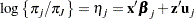and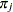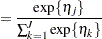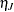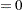As before, suppose that the two conditions to be compared are identified with subscriptsand. The log odds ratio of outcomeversusfor the two conditions is then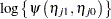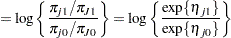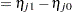Note that the log odds ratios are again differences on the scale of the linear predictor, but they depend on the response category. The GLIMMIX procedure determines the estimable functions whose differences represent log odds ratios as discussed previously but produces separate estimates for each nonreference response category.

In models for ordinal data, PROC GLIMMIX models the logits of cumulative probabilities. Thus, the estimates on the linear scale represent log cumulative odds. The cumulative logits are formed as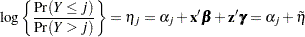so that the linear predictor depends on the response category only through the intercepts (cutoffs)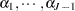. The odds ratio comparing two conditions represented by linear predictors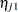and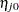is then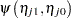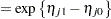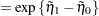and is independent of category.